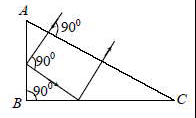# Cat A Sample Paper Set III For Class Ix And X

20 Questions | Attempts: 723Settings• 1.
A ray of light falls on a prism ABC (AB = BC) and travels as shown in figure. The refractive index of the prism material should be greater than
• A.

4/3

• B.
• C.

1.5

• D.
• 2.
A radioactive sample has N0 active atoms at t = 0. If the rate of disintegration at any time is R and the number of atoms is N, then the ratio R/N varies with time as
• A.
• B.
• C.
• D.
• 3.
The wavelength of K     X-rays for lead isotopes  , respectively. Then
• A.
• B.
• C.
• D.
• 4.
For a particle executing simple harmonic motion, the kinetic energy k is given by, k = k0Cos2  (where  is constant). The maximum value of potential energy is
• A.
• B.

Zero

• C.
• D.
• 5.
Which one of the following gases is adsorbed most by activated charcoal?
• A.

N2

• B.

Cl2

• C.

CO2

• D.

O2

• 6.
Electrolytic reduction method is used in the extraction of
• A.

Noble metal

• B.

Transition metal

• C.

Highly electropositive elements

• D.

Highly electronegative elements

• 7.
How many grams of invert sugar can be produced by the hydrolysis of 1.00 g of sucrose, C12H22O11 ?
• A.

1.00 g

• B.

2.00 g

• C.

1.05 g

• D.

0.50 g

• 8.
A transverse wave is travelling along a string from left to right. The figure below represents the shape of the string at a given instant. At this instant, among the following, choose the wrong statement
• A.

Points D, E, F have velocity in + y direction

• B.

Points A, B and H have velocity in – y direction

• C.

Points C and G have zero velocity

• D.

Point A and E have minimum velocity

• 9.
Arrange the following electrolytes in increasing order of coagulation power for 23 As S colloid.
• A.

(A1) < (A2) < (A3)

• B.

(A1) > (A2) > (A3)

• C.

(A3) < (A1) < (A2)

• D.

(A2) < (A3) < (A1)

• 10.
If A is any invertible idempotent matrix and B = 7A7 + 6A6 + 5A5 + ..... A, then det (B) is equal to
• A.

15 det (A)

• B.

20 det (A)

• C.

28 det (A)

• D.

30 det (A)

• 11.
In which of the following metal carbonyl the C–O bond is the weakest?
• A.
• B.
• C.
• D.
• 12.
If in a ABC, a = 6, b = 3 and cos (A – B) =4/5 , then
• A.
• B.
• C.

Area ( ABC) = 10 sq. units

• D.

Area ( ABC)= 18 sq. units

• 13.
Seven digits from the digits 1, 2, 3, 4, 5, 6, 7, 8 and 9 are picked and a number is formed randomly then the probability that the number is divisible by 9 is PARAGRAPH An equilateral triangle ABC is inscribed into a circle of radius ‘R’. Another circle is inscribed into the triangle ABC and a square is inscribed into the incircle of the triangle
• A.

3/7

• B.

1/9

• C.

27/36

• D.

None of these

• 14.
Area of square PQRS is
• A.
• B.
• C.
• D.

None of these

• 15.
The length of side of equilateral triangle ABC is
• A.

R

• B.
• C.
• D.

None of these

• 16.
Lateralmeristemis responsible for
• A.

Growth in parenchyma

• B.

Growth in thickness

• C.

Growth in cortex

• D.

Growth in length

• 17.
What is a characteristic of archaebacteria?
• A.

Most are disease – causing

• B.

• C.

They use sun to produce food and energy

• D.

Theythrive in extreme environment

• 18.
Which of the following statements are true about Endoplasmic Reticulum? (1)Smooth endoplasmic reticulum makes lipids (2) It is also called the control centre of the cell (3) It processes carbohydrates (4) Itmodifies chemicals that are toxic to the cell
• A.

(1), (2) and (3)

• B.

(1), (2) and (4)

• C.

Only (1) and (4)

• D.

(1), (3) and (4)

• 19.
If pteridophytes had this feature, theywould be placed in the same group as gymnosperms.
• A.

Seed bearing capacity

• B.

Plant bodydifferentiated into root, stem, and leaves

• C.

Vascular tissues

• D.

Well differentiated reproductive tissue

• 20.
Xerophtalmia is caused due to the deficiency of
• A.

VitaminC

• B.

VitaminB

• C.

VitaminA

• D.

VitaminD

## Related TopicsBack to top
×

Wait!
Here's an interesting quiz for you.